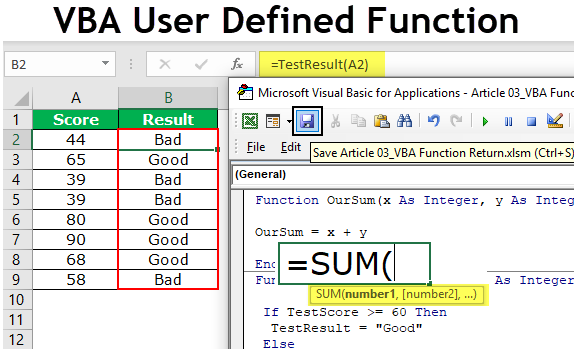# How to Create User Defined Function In Excel

Oct 16, 2020 • edited Oct 19, 2020Below we will look at a program in Excel VBA that creates a User Defined Function. Excel has a large collection of functions. In most situations those functions are sufficient to get the job done. If not, you can create your own function called User Defined Function or custom Excel function. You can access a User Defined Function just like any other Excel function.

We want to create a function called SUMEVENNUMBERS that finds the sum of the even numbers of a randomly selected range.

Situation:User defined functions need to be placed into a module.

1. Open the Visual Basic Editor and click Insert, Module.

2. Add the following code line:

Function SUMEVENNUMBERS(rng As Range)

The name of our Function is SUMEVENNUMBERS. The part between the brackets means we give Excel VBA a range as input. We name our range rng, but you can use any name.

3. Next, we declare a Range object and call it cell.

``````          Dim cell As Range
``````

4. We want to check each cell in a randomly selected range (this range can be of any size). In Excel VBA, you can use the For Each Next loop for this. Add the following code lines:

For Each cell In rng

Next cell

Note: cell is randomly chosen here, you can use any name.

5. Next, we check for each value in this range whether it is even or not. We use the Mod operator for this. The Mod operator gives the remainder of a division. So 7 mod 2 would give 1. 7 is divided by 2 (3 times) to give a remainder of 1. Having said this, it is easy to check whether a number is even or not. Only if the remainder of a number divided by 2 is 0, the number is even. 8 mod 2 gives 0, 8 is divided by 2 exactly 4 times, and therefore 8 is even. Add the following If statement to the For Each Next loop.

If cell.Value Mod 2 = 0 Then

End If

6. Only if this statement is true, we add the value to SUMEVENNUMBERS. Add the following code line in the If statement.

``````          SUMEVENNUMBERS = SUMEVENNUMBERS + cell.Value
``````

7. Don't forget to end the function (outside the loop).

End Function

8. Now you can use this function, just like any other Excel function, to find the sum of the even numbers of a randomly selected range.

Result:Well done! That's how easy User Defined Functions in Excel VBA are. Note: this function is only available in this workbook.

#Tutorial#How To#VBA#Function and Sub

How to use spin buttons in Excel VBA

Excel VBA - Union And Intersect